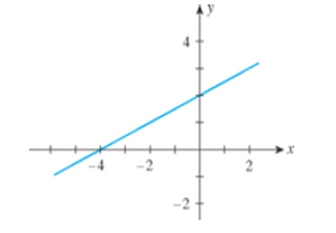Chapter 1.4, Problem 7E

Chapter
Section
Textbook Problem

In Exercises 7-10, find the slope of the line shown in each figure.7.To determine

To find: The slope of the line of the given figure.

Explanation

The graph shows the slope of line,

Figure (1)

The two points on the graph are (0,2) and (4,0) .

The slope of the equation of line is calculated as,

m=y2y1x2x1 (1)

Substitute 0 for y2

Still sussing out bartleby?

Check out a sample textbook solution.

See a sample solution

The Solution to Your Study Problems

Bartleby provides explanations to thousands of textbook problems written by our experts, many with advanced degrees!

Get Started

Find the inverse function of f(x)x2+2forx0.

Calculus: An Applied Approach (MindTap Course List)

Place the following set of n = 20 scores in a frequency distribution table.

Essentials of Statistics for The Behavioral Sciences (MindTap Course List)

In problems 49-54, rationalize the denominator and then simpify. 52.

Mathematical Applications for the Management, Life, and Social Sciences

Elementary Technical Mathematics

In Exercises 21-26, use the laws of logic to prove the propositions. p(pq)pq

Finite Mathematics for the Managerial, Life, and Social Sciences

If f(x) = 2e3x, f(x) = a) 2e3x b) 2xe3x c) 6e3x d) 6xe3x

Study Guide for Stewart's Single Variable Calculus: Early Transcendentals, 8th

Rewritten in reverse order,

Study Guide for Stewart's Multivariable Calculus, 8th

Self Check Expand: (pq)3.

College Algebra (MindTap Course List)